## Kolmogorov-Arnold-Moser Theorem

A theorem outlined in 1954 by Kolmogorov which was subsequently proved in the 1960s by Arnold and Moser (Tabor 1989, p. 105). It gives conditions under which Chaos is restricted in extent. Moser's 1962 proof was valid for Twist Maps(1)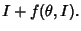(2)

In 1963, Arnold produced a proof for Hamiltonian systems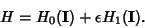(3)

The original theorem required perturbations, although this has since been significantly increased. Arnold's proof required, and Moser's original proof required. Subsequently, Moser's version has been reduced to, then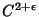, although counterexamples are known for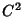. Conditions for applicability of the KAM theorem are:
1. small perturbations,

2. smooth perturbations, and

3. sufficiently irrational Winding Number.

Moser considered an integrable Hamiltonian functionwith a Torus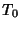and set of frequencies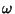having an incommensurate frequency vector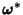(i.e.,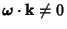for all Integers). Letbe perturbed by some periodic function. The KAM theorem states that, ifis small enough, then for almost every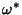there exists an invariant Torusof the perturbed system such thatis close to''. Moreover, the Toriform a set of Positive measures whose complement has a measure which tends to zero as. A useful paraphrase of the KAM theorem is, For sufficiently small perturbation, almost all Tori (excluding those with rational frequency vectors) are preserved.'' The theorem thus explicitly excludes Tori with rationally related frequencies, that is,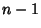conditions of the form(4)

These Tori are destroyed by the perturbation. For a system with two Degrees of Freedom, the condition of closed orbits is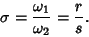(5)

For a Quasiperiodic Orbit,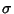is Irrational. KAM shows that the preserved Tori satisfy the irrationality condition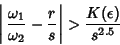(6)

for alland, although not much is known about.

The KAM theorem broke the deadlock of the small divisor problem in classical perturbation theory, and provides the starting point for an understanding of the appearance of Chaos. For a Hamiltonian System, the Isoenergetic Nondegeneracy condition(7)

guarantees preservation of most invariant Tori under small perturbations. The Arnold version states that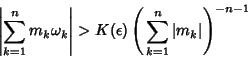(8)

for all. This condition is less restrictive than Moser's, so fewer points are excluded.